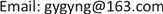1. 引言

2. 图像获取及预处理2.1. 实验图像获取

2.2. 图像预处理

3. 算法实现3.1. 图像相关性检测

r k = ∑ m ∑ n ( A k , m n − A k ¯ ) ( A k − 1 , m n − A k − 1 ¯ ) ( ∑ m ∑ n ( A k , m n − A k ¯ ) 2 ) ( ∑ m ∑ n ( A k − 1 , m n − A k − 1 ¯ ) 2 ) A ¯ (1)

r k = ∑ m ∑ n ( A k , m n − A k ¯ ) ( A k − 1 , m n − A k − 1 ¯ ) ( ∑ m ∑ n ( A k , m n − A k ¯ ) 2 ) ( ∑ m ∑ n ( A k − 1 , m n − A k − 1 ¯ ) 2 ) (2)

3.2. 图像边缘信息

3.3. 图像频域能量

3.4. 图像信息熵

H = − ∑ k = 1 256 p ( k ) × log 2 p ( k ) (3)

4. 结论

1) 使用相关系数法、边缘信息、频域能量和平均信息熵都可以将清晰图像序列和脏污图像序列区分开。脏污图像序列中的图像相关性大于清晰图像序列中的图像相关性；清晰图像序列中的边缘信息高于脏污图像序列中的边缘信息；清晰图像序列中的频域能量高于脏污图像序列中的频域能量；清晰图像序列的平均信息熵高于脏污图像序列的平均信息熵。

2) 在图像尺寸相同的情况下，平均信息熵计算速度最快，有较高的应用价值。